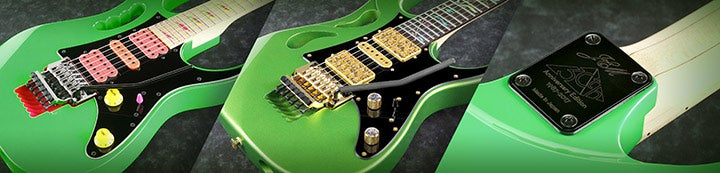1 - 2 of 2 Posts

#### theapprentice

·
##### Registered
Joined
·
197 Posts
Discussion Starter · ·
I'm not entirely sure if this is useful, but it'll be here if you guys would need it.

For example, a sextuplet lick in 120 is actually the same speed as a 4 note per beat lick in 180bpm. How did I come up with that?

Here's how:

1.) Determine your reference tempo and note grouping which you'd like to use.
2.) Take the tempo and divide 60 (again, the number of seconds per minute) by it.
3.) Take the quotient and divide it by the number of notes per beat that you chose from number 1.
4.) Now, determine the number of notes per beat that you'd like to know the tempo for. Multiply that to the answer from number 3.
5.) Take that product and divide 60 by it.

Example:
1.) 160 bpm, 4 notes per beat
2.) 60/160 = 0.375
3.) 0.375/4 = 0.09375
4.) I'd like to find out the tempo for sextuplets at the same speed, so: 0.09375*6 = 0.5625
5.) 60/0.5625 = 106.66 bpm

This would probably be practical for when you would like to determine what kinds of interesting note groupings and sequences you could use for a particular solo at a given tempo. For example, at about 120bpm, 4 note per beat runs would probably sound a bit monotonous, but when you use a couple of sextuplet runs in between some of those normal ones the solo would probably have a better variety of textures tempo-wise.

#### exutus

·
##### Banned
Joined
·
42 Posts
Why don't you write it as a program, that would be simple for you to calculate after.

I wrote a program to calculate differant delays (MS) after you enter the tempo in bpm. It really saves some time!

Edit: like for a ghost note delay when playing at the speed of 4 notes per bar, divide 45 000 by the tempo.

45000/bpm.
for instance; 45000/137bpm=328,46
Set the delay to 328 miliseconds.

Do I don't have to do the equation everytime1 - 2 of 2 Posts